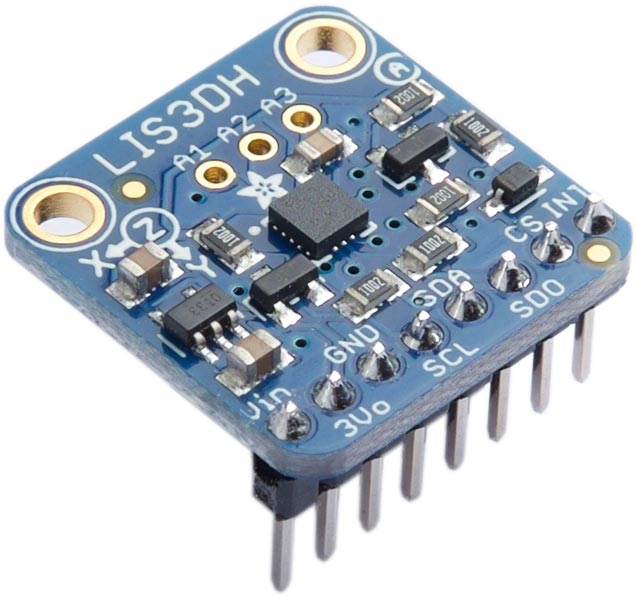## LIS3DH 3-Axis Accelerometer

The LIS3DH  is a low power triple-axis accelerometer with 10-bit precision, selectable scaling, and both I2C and SPI interfaces. It operates from 1.71V to 3.6V, so you'll need a regulator and logic-level conversion if you want to use it on a 5V board.

A breakout is available from Adafruit :To use I2C mode the CS pin must be tied high. On the Adafruit board it defaults to I2C if left disconnected.

In I2C mode there are two possible addresses, selected by the SDO pin. By default the address is 0x18; tie SDO high for 0x19.

The sensor provides a number of different operating modes for different applications, and a FIFO allowing you to buffer 32 10-bit readings. The following routines run the sensor in the default Normal Mode, which provides 10-bit accuracy, and FIFO Bypass mode, which ignores the FIFO.

#### Setting the sample rate

By default the sensor is in low-power mode, so to take readings you need to set the rate. The following routines lis3dh-rate takes a parameter from 0 to 9:

```(defun lis3dh-rate (x)
(with-i2c (s #x18)
(write-byte #x20 s)
(write-byte (logior (ash x 4) 7) s)))```

For example to set a 10Hz sample rate:

`(lis3dh-rate 2)`

The following routine s16 returns a signed 16-bit integer from two bytes, LSB first:

```(defun s16 (s)
(- d (ash (logand d #x8000) 1))))```

The following routine lis3dh-xyz then returns the acceleration data as a list of three signed integers:

```(defun lis3dh-xyz ()
(with-i2c (s #x18)
(write-byte (+ #x28 #x80) s) ; Set top bit to read multiple bytes
(restart-i2c s 6)
(let (dat)
(dotimes (i 3) (push (s16 s) dat))
(reverse dat))))```

At the default full-scale sensitivity, 2g, a value of 32767 represents 2g and -32768 represents -2g.

For example:

`> (lis3dh-xyz)(256 704 16768)`

If the sensor is stationary the x and y figures will be close to zero, and the z value will be close to 16384, corresponding to 1g.

#### Floating-point version

If you're running on a 32-bit version of uLisp with floating-point, the following routine will return the values in g; it reads the currently selected full-scale sensitivity:

```(defun lis3dh-g3d ()
(let ((fs (with-i2c (s #x18)
(write-byte #x23 s)
(restart-i2c s 1)
(logand 3 (ash (read-byte s) -4)))))
(mapcar (lambda (i) (/ i (ash 16384 (- fs)))) (lis3dh-xyz))))```

For example:

`> (lis3dh-g3d)(0.0273438 0.0507813 1.02734)`

#### Setting the sensitivity

The following routine set sets the full-scale sensitivity of the accelerometer:

```(defun lis3dh-set (x)
(with-i2c (s #x18)
(write-byte #x23 s)
(write-byte (ash x 4) s)))```

The parameter can be 0 for ±2g, 1 for ±4g, 2 for ±8g, or 3 for ±16g.

1. ^ LIS3DH Datasheet on ST.com.